## What is Telescoping Sum?

Telescoping sum is one of very useful summation properties. Telescoping sum simplify our summation because it says that sum of a difference is equal to the last and first term only, not depending on the middle terms.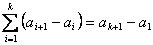ExampleExample

Suppose we have a sequence of numbers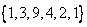then we can put index on the sequence such that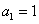,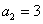,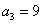,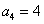,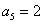,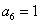and we want to compute the sum of difference< Previous | Next | Contents >